##### Pre-Calculus For DummiesCosecant is almost exactly the same as secant because it's the reciprocal of sine (as opposed to cosine). Anywhere sine has a value of 0, you see an asymptote in the cosecant graph. Because the sine graph crosses the x-axis three times on the interval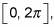you have three asymptotes and two sub-intervals to graph.

The reciprocal of 0 is undefined, and the reciprocal of an undefined value is 0. Because the graph of sine is never undefined, the reciprocal of sine can never be 0. For this reason, the parent graph of the cosecant function f(x) = csc x has no x-intercepts, so don't bother looking for them.

The following steps explain how to graph cosecant:

1. Find the asymptotes of the graph.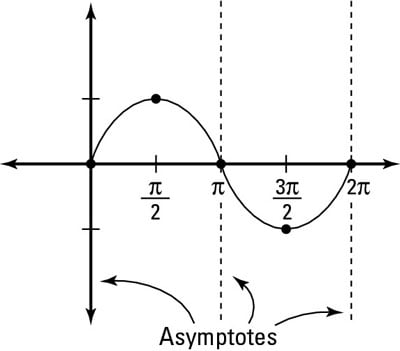The graph of sine reveals the asymptotes of cosecant.

Because cosecant is the reciprocal of sine, any place on sine's graph where the value is 0 creates an asymptote on cosecant's graph. The parent graph of sine has values of 0 at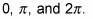So cosecant has three asymptotes. The figure shows these asymptotes.

2. Calculate what happens to the graph at the first interval between 0 and pi.

The period of the parent sine graph starts at 0 and ends at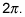You can figure out what the graph does in between the first asymptote at 0 and the second asymptote at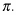The graph of sine goes from 0 to 1 and then back down again. Cosecant takes the reciprocal of these values, which causes the graph to get bigger.

3. Repeat for the second interval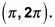If you refer to the sine graph, you see that it goes from 0 down to –1 and then back up again. Because cosecant is the reciprocal, its graph gets bigger in the negative direction.

4. Find the domain and range of the graph.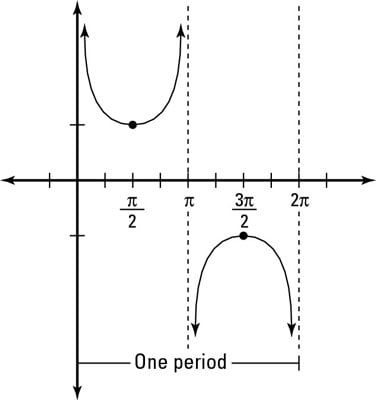Cosecant's asymptotes start at 0 and repeat every pi. Its domain is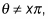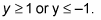Its range, therefore, is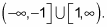You can see the full parent graph,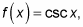in the figure.